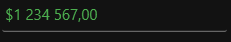# Getting Started with WPF Currency TextBox

18 Oct 20229 minutes to read

This section explains how to create a WPF `CurrencyTextBox` control and its features.

## Assembly deployment

Refer to the control dependencies section to get the list of assemblies or NuGet package that needs to be added as a reference to use the control in any application.

You can find more details about installing the NuGet package in a WPF application in the following link:

How to install nuget packages

## Adding WPF CurrencyTextBox via designer

You can add the CurrencyTextBox control to an application by dragging it from the toolbox to a view of the designer. The following dependent assembly will be added automatically:

• Syncfusion.Shared.WPF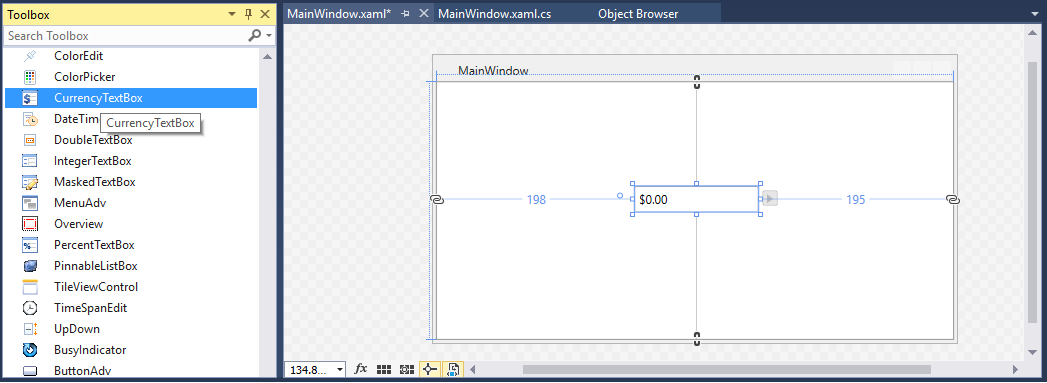## Adding WPF CurrencyTextBox via XAML

1. Create a new WPF project in Visual Studio.

2. Add the Syncfusion.Shared.WPF assembly references to the project.

3. Import Syncfusion WPF schema http://schemas.syncfusion.com/wpf and declare the `CurrencyTextBox` control in XAML page.

``````<Window xmlns="http://schemas.microsoft.com/winfx/2006/xaml/presentation"
xmlns:x="http://schemas.microsoft.com/winfx/2006/xaml"
xmlns:syncfusion="http://schemas.syncfusion.com/wpf"
x:Class="CurrencyTextBoxSample.MainWindow"
Title="CurrencyTextBox Sample" Height="350" Width="525">
<Grid>
<syncfusion:CurrencyTextBox x:Name="currencyTextBox" Width="100" Height="25" VerticalAlignment="Center" HorizontalAlignment="Center"/>
</Grid>
</Window>``````

## Adding WPF CurrencyTextBox via C#

1. Create a new WPF application via Visual Studio.

2. Add the Syncfusion.Shared.WPF assembly references to the project.

3. Include the required namespace.

``using Syncfusion.Windows.Shared;``
4. Create an instance of CurrencyTextBox and add it to the window.

``````//Creating an instance of CurrencyTextBox control

CurrencyTextBox currencyTextBox = new CurrencyTextBox();

// Setting height and width to CurrencyTextBox

currencyTextBox.Height = 25;
currencyTextBox.Width = 100;

this.Content = currencyTextBox;``````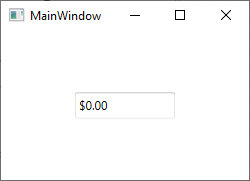## Setting Value

The value of the `CurrencyTextBox` can be set by using the Value property.

``<syncfusion:CurrencyTextBox x:Name="currencyTextBox" Width="100" Height="23" Value="100"/>``
``````CurrencyTextBox currencyTextBox = new CurrencyTextBox();
currencyTextBox.Width = 100;
currencyTextBox.Height = 23;
currencyTextBox.Value = 100;``````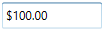NOTE

Do not use the Text property to set the value for the CurrencyTextBox. Use only the `Value` property.

### Binding Value

Data binding is the method of forming a connection between the application UI and business logic. Data binding can be unidirectional (source -> target or target <- source) or bidirectional (source <-> target). You can bind data to the `CurrencyTextBox` using the `Value` Property.

The following code snippets illustrate the value binding from one `CurrencyTextBox` to another.

``````<StackPanel>
<syncfusion:CurrencyTextBox x:Name="currencyTextBox1" Height="25" Width="100" Value="{Binding MyValue,UpdateSourceTrigger=PropertyChanged}"/>
<syncfusion:CurrencyTextBox x:Name="currencyTextBox2" Width="100" Height="25" Value="{Binding MyValue,UpdateSourceTrigger=PropertyChanged}" />
</StackPanel>``````

ViewModel.cs

``````class ViewModel : NotificationObject
{
private double myValue;
public double MyValue
{
get
{
return myValue;
}
set
{
myValue = value;
RaisePropertyChanged("MyValue");
}
}
}``````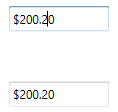The `CurrencyTextBox` control can notifies the value changes through the ValueChanged event. You can get old value and new Value from `OldValue` and `NewValue` properties in `ValueChanged` event.

``<syncfusion:CurrencyTextBox ValueChanged="CurrencyTextBox_ValueChanged"/>``
``````CurrencyTextBox currencyTextBox = new CurrencyTextBox();
currencyTextBox.ValueChanged += new PropertyChangedCallback(CurrencyTextBox_ValueChanged);``````

You can handle the event as follows:

``````private void CurrencyTextBox_ValueChanged(DependencyObject d, DependencyPropertyChangedEventArgs e)
{
// Get old and new value
var newValue = e.NewValue;
var oldValue = e.OldValue;
}``````

## Min Max Value Restriction

The `Value` of `CurrencyTextBox` can be restricted within maximum and minimum limit. You can define the minimum and maximum values by setting the MinValue and MaxValue properties. It allows the user to enter the value between `MinValue` and `MaxValue`.

``<syncfusion:CurrencyTextBox x:Name="currencyTextBox" Width="100" Height="25" Value="455" MaxValue="999.99" MinValue="-999.99"/>``
``````CurrencyTextBox currencyTextBox = new CurrencyTextBox();
currencyTextBox.Width = 100;
currencyTextBox.Height = 25;
//Setting minimum value
currencyTextBox.MinValue = -999.99;
//Setting maximum value
currencyTextBox.MaxValue = 999.99;
currencyTextBox.Value = 455;``````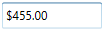## Step Interval to increase or decrease the value

The `CurrencyTextBox` control allows to increase or decrease the value by pressing up and down arrow keys in keyboard or mouse wheel over the control. The ScrollInterval property is used to specify the increment or decrement intervals. The default value of `ScrollInterval` is 1.

``````<syncfusion:CurrencyTextBox x:Name="currencyTextBox" Width="100" Height="25" Value="8"
IsScrollingOnCircle="True" ScrollInterval="4"/>``````
``````CurrencyTextBox currencyTextBox = new CurrencyTextBox();
currencyTextBox.Width = 100;
currencyTextBox.Height = 25;
currencyTextBox.MinValue = 0;
currencyTextBox.MaxValue = 100;
currencyTextBox.Value = 8;
currencyTextBox.IsScrollingOnCircle = true;
currencyTextBox.ScrollInterval = 4;``````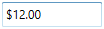## Formatting the value

You can customize the number format by using either the NumberFormat property or the CurrencyGroupSeparator, CurrencyGroupSizes, CurrencyDecimalDigits and CurrencyDecimalSeparator, CurrencySymbol, CurrencyNegativePattern, and CurrencyPositivePattern properties of CurrencyTextBox.

``````<syncfusion:CurrencyTextBox x:Name="currencyTextBox" Height="25" Width="150"  Value="1234567">
<syncfusion:CurrencyTextBox.NumberFormat >
<numberformat:NumberFormatInfo CurrencyGroupSeparator="/" CurrencyDecimalDigits="4" CurrencyDecimalSeparator="*"   CurrencySymbol="\$"/>
</syncfusion:CurrencyTextBox.NumberFormat>
</syncfusion:CurrencyTextBox>``````
``````CurrencyTextBox currencyTextBox = new CurrencyTextBox();
currencyTextBox.Width = 100;
currencyTextBox.Height = 25;
currencyTextBox.Value = 1234567;
currencyTextBox.NumberFormat = new NumberFormatInfo()
{
CurrencyGroupSeparator = "/",
CurrencyDecimalDigits = 4,
CurrencyDecimalSeparator = "*",
CurrencySymbol = "\$"
};``````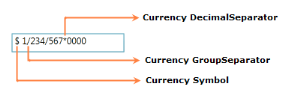## Setting the Culture

The `CurrencyTextBox` provides support for globalization by using the Culture property. The `Culture` is used to format the decimal separator and group separator of the `CurrencyTextBox` value based on the respective culture.

``<syncfusion:CurrencyTextBox x:Name="currencyTextBox" Height="25" Width="100" Culture="en-US" Value="1234567"/>``
``````CurrencyTextBox currencyTextBox = new CurrencyTextBox();
currencyTextBox.Width = 100;
currencyTextBox.Height = 25;
currencyTextBox.Value = 1234567;
currencyTextBox.Culture = new System.Globalization.CultureInfo("en-US");``````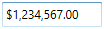NOTE

When you use both `NumberFormat` and `Culture`, the `NumberFormat` will have a higher priority.

## Theme

CurrencyTextBox supports various built-in themes. Refer to the below links to apply themes for the CurrencyTextBox,